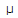# Electronics - Capacitors - Discussion

### Discussion :: Capacitors - Filling the Blanks (Q.No.1)

1.

If a 5 V, 60 Hz voltage is applied to a 4.7F capacitor, the circuit current will be ______ .

 [A]. 0.886 mA [B]. 8.86 mA [C]. 8.86A [D]. 88.6A

Explanation:

No answer description available for this question.

 Sumit said: (Dec 21, 2011) I = v/Xc

 Savitha U R said: (Feb 16, 2012) Xc=1/2*pi*f*c=1/2*3.14*60*4.7*10^-6 Xc=1/1770.96*10^-6 =564.665 I=V/Xc=5/564.665 =0.008854=8.85*101^-3=8.85mA